# Thermodynamics Heat and Temperature Thermal Properties of Matter

• Slides: 46
Download presentationThermodynamics Heat and Temperature, Thermal Properties of Matter, The First Law of Thermodynamics, The Second Law of Thermodynamics, Heat Engines, Internal-Combustion Engines, Refrigerators, Carnot Cycle, Entropy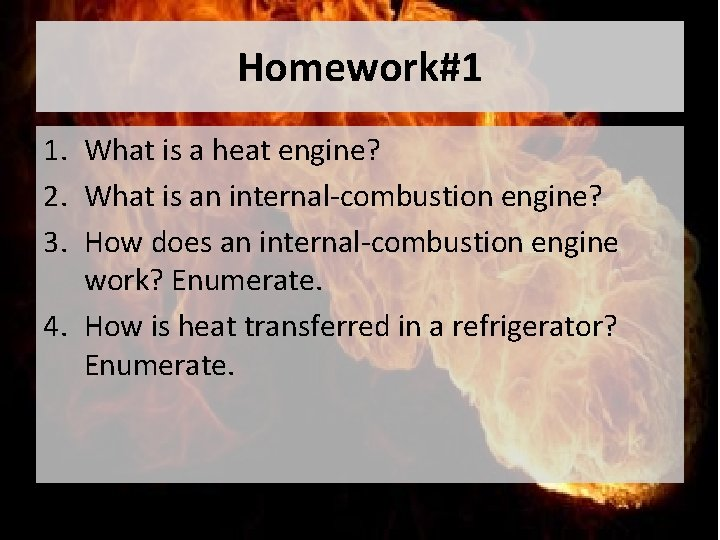Homework#1 1. What is a heat engine? 2. What is an internal-combustion engine? 3. How does an internal-combustion engine work? Enumerate. 4. How is heat transferred in a refrigerator? Enumerate.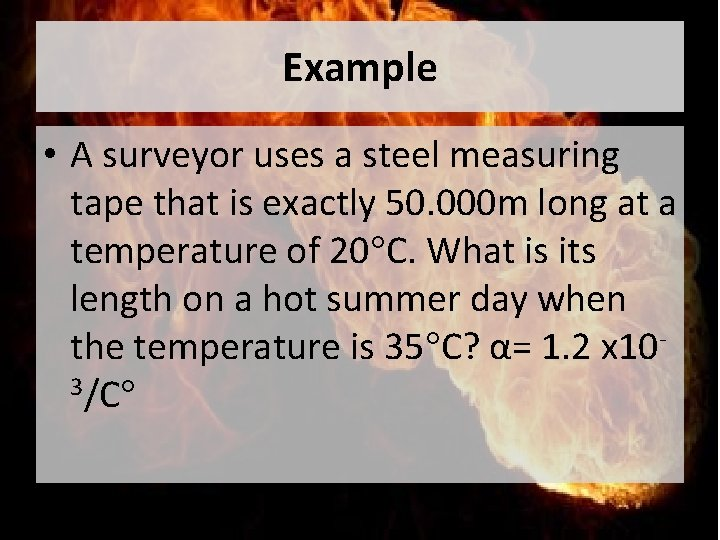Example • A surveyor uses a steel measuring tape that is exactly 50. 000 m long at a temperature of 20°C. What is its length on a hot summer day when the temperature is 35°C? α= 1. 2 x 103/C°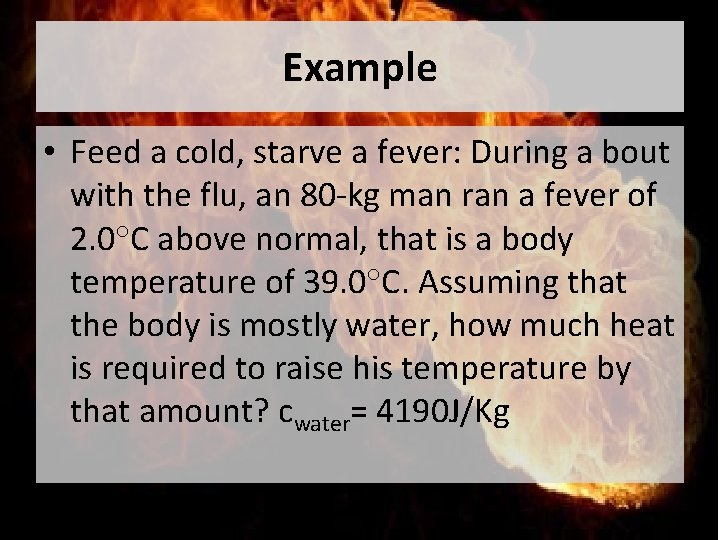Example • Feed a cold, starve a fever: During a bout with the flu, an 80 -kg man ran a fever of 2. 0°C above normal, that is a body temperature of 39. 0°C. Assuming that the body is mostly water, how much heat is required to raise his temperature by that amount? cwater= 4190 J/Kg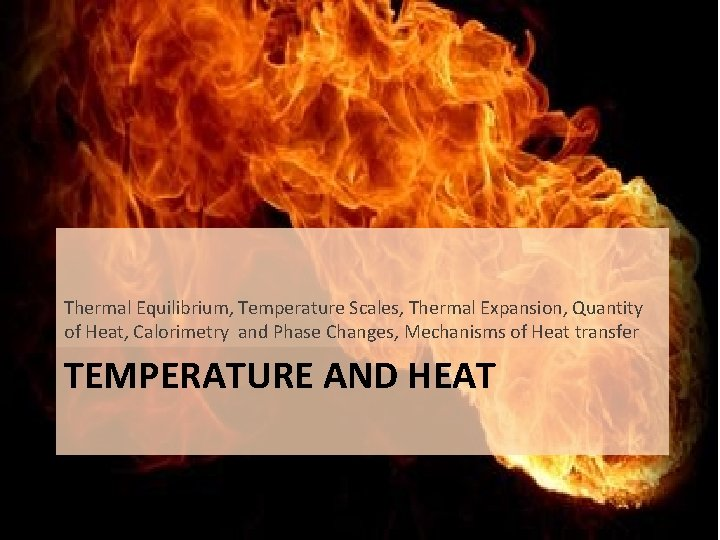Thermal Equilibrium, Temperature Scales, Thermal Expansion, Quantity of Heat, Calorimetry and Phase Changes, Mechanisms of Heat transfer TEMPERATURE AND HEATTemperature and Thermal Equilibrium • Temperature is a measure of hotness or coldness. • When an interaction causes no further change in the system, then it is in the state of thermal equilibrium. • Two systems are in thermal equilibrium if and only if they have the same temperature.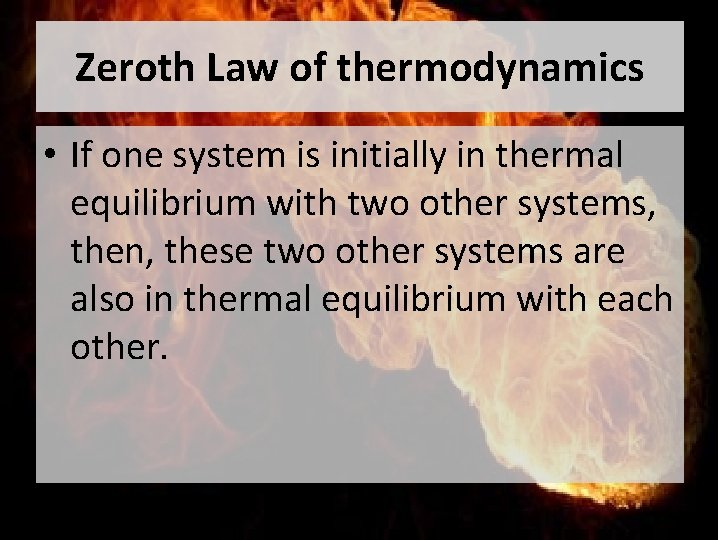Zeroth Law of thermodynamics • If one system is initially in thermal equilibrium with two other systems, then, these two other systems are also in thermal equilibrium with each other.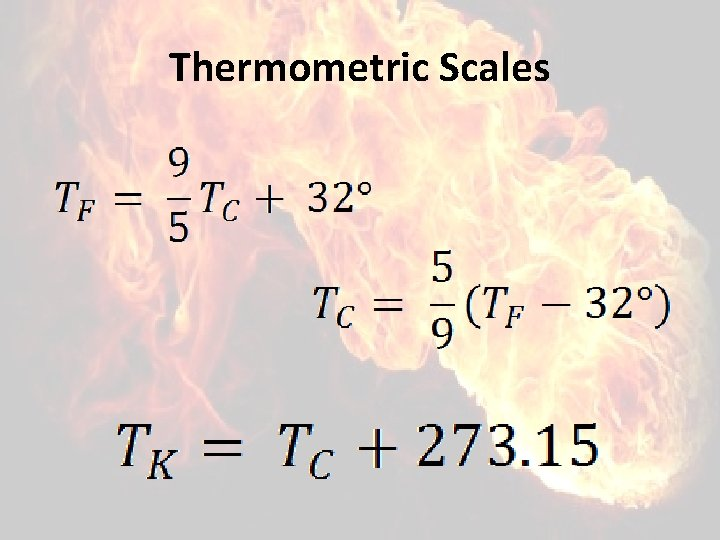Thermometric Scales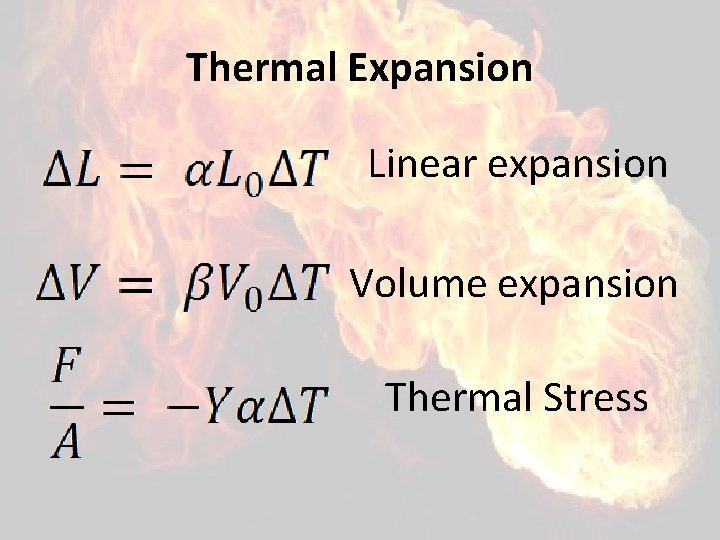Thermal Expansion Linear expansion Volume expansion Thermal Stress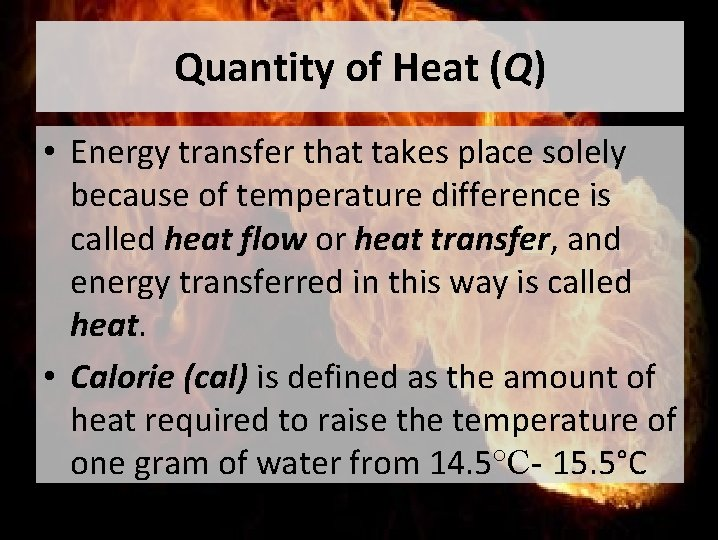Quantity of Heat (Q) • Energy transfer that takes place solely because of temperature difference is called heat flow or heat transfer, and energy transferred in this way is called heat. • Calorie (cal) is defined as the amount of heat required to raise the temperature of one gram of water from 14. 5°C- 15. 5°C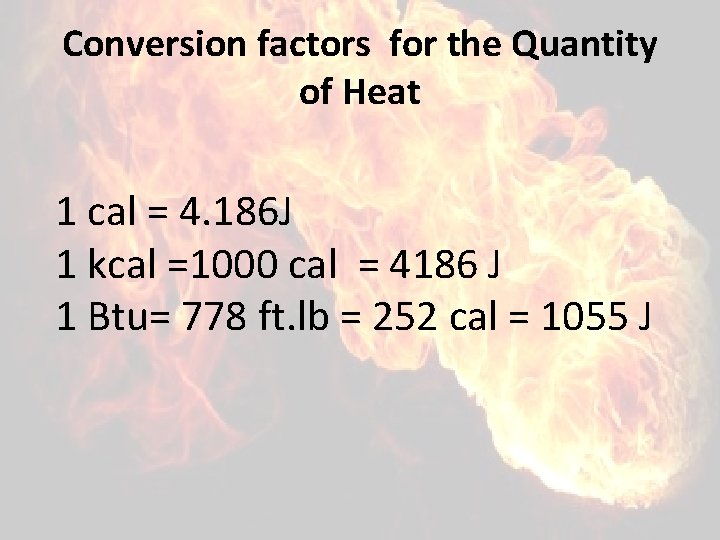Conversion factors for the Quantity of Heat 1 cal = 4. 186 J 1 kcal =1000 cal = 4186 J 1 Btu= 778 ft. lb = 252 cal = 1055 J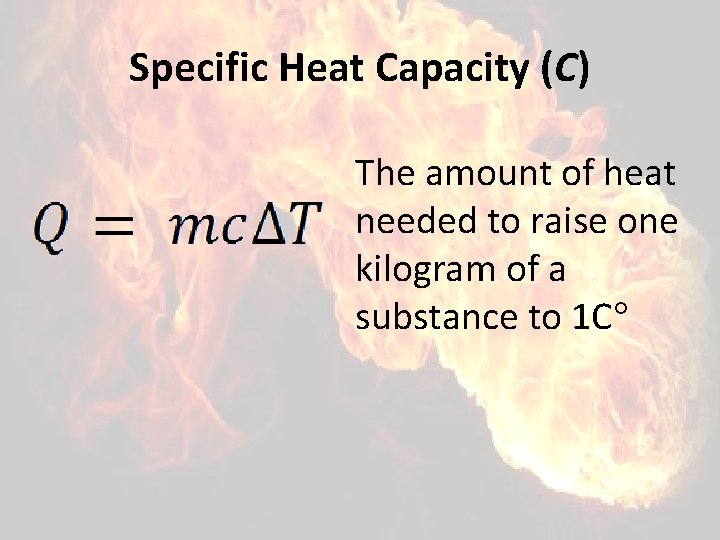Specific Heat Capacity (C) The amount of heat needed to raise one kilogram of a substance to 1 C°Phase Changes • The term phase is used to describe the specific state of matter. • A transition from one phase to another is called a phase change or phase transition.Phase Change • Heat of fusion (Lf)- the heat required per unit mass in changing solid to liquid • Heat of vaporization (Lv)- the heat required per unit mass in changing liquid to gas • Heat of combustion (Lc) - the heat required per unit mass in complete combustion of one gram of gasolineMechanisms of Heat transfer • Conduction occurs between a body or between two bodies in contact. • Convection depends on motion of mass from one region of space to another. • Radiation is heat transfer by EM radiation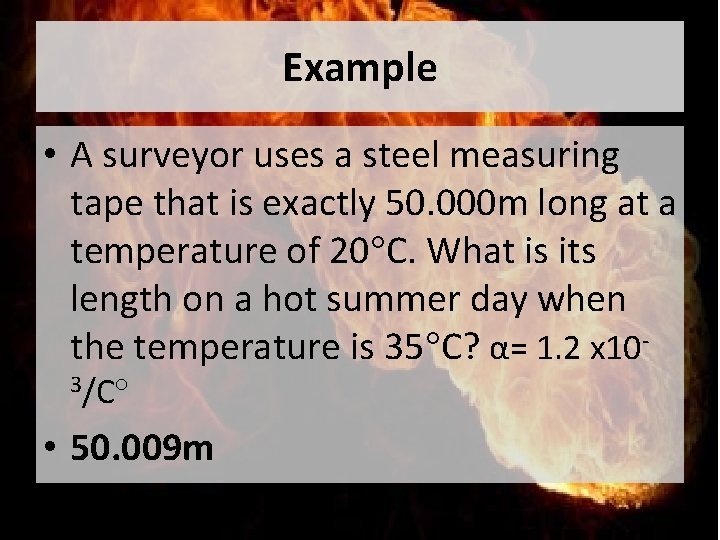Example • A surveyor uses a steel measuring tape that is exactly 50. 000 m long at a temperature of 20°C. What is its length on a hot summer day when the temperature is 35°C? α= 1. 2 x 103/C° • 50. 009 m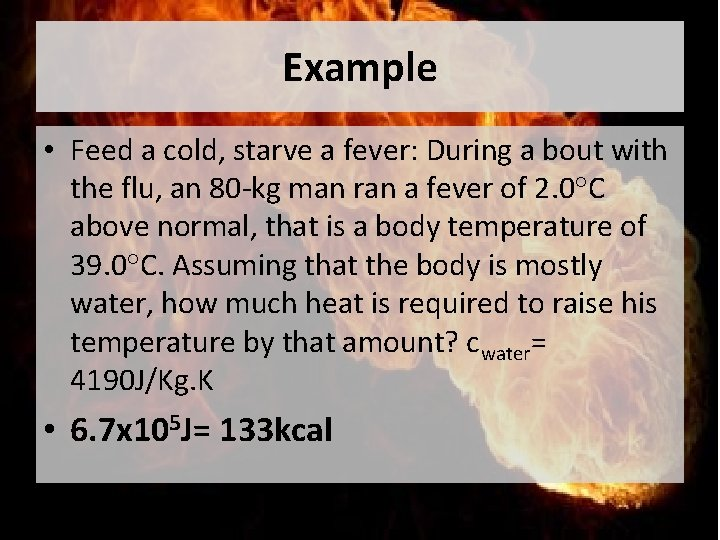Example • Feed a cold, starve a fever: During a bout with the flu, an 80 -kg man ran a fever of 2. 0°C above normal, that is a body temperature of 39. 0°C. Assuming that the body is mostly water, how much heat is required to raise his temperature by that amount? cwater= 4190 J/Kg. K • 6. 7 x 105 J= 133 kcalMolecular Properties of Matter, KMT, Heat Capacities, Phases of Matter THERMAL PROPERTIES OF MATTERKMT 1. Gases are considered to be composed of minute discrete particles. 2. The molecule in a container are believed to be in ceaseless motion during which they collide with each other and the walls of the container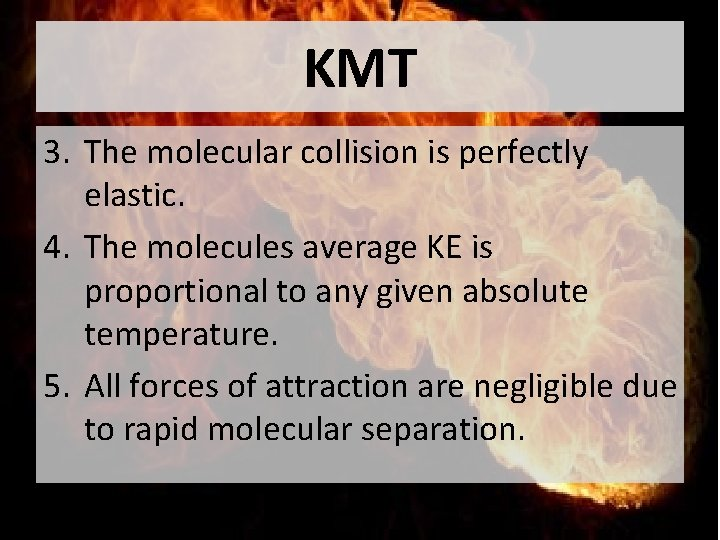KMT 3. The molecular collision is perfectly elastic. 4. The molecules average KE is proportional to any given absolute temperature. 5. All forces of attraction are negligible due to rapid molecular separation.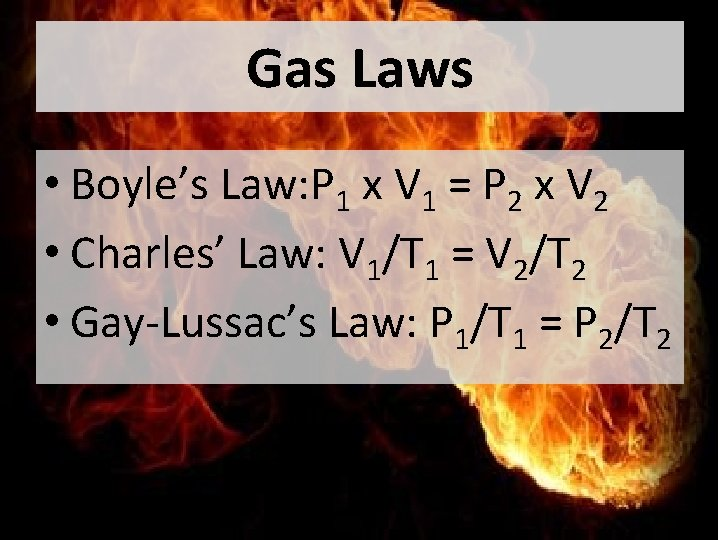Gas Laws • Boyle’s Law: P 1 x V 1 = P 2 x V 2 • Charles’ Law: V 1/T 1 = V 2/T 2 • Gay-Lussac’s Law: P 1/T 1 = P 2/T 2Ideal Gas law • Emphasizes on the amount of substance and its effect on the volume of a gas, represented by Avogadro’s law • nα V if PVα RT, then PVα n. RT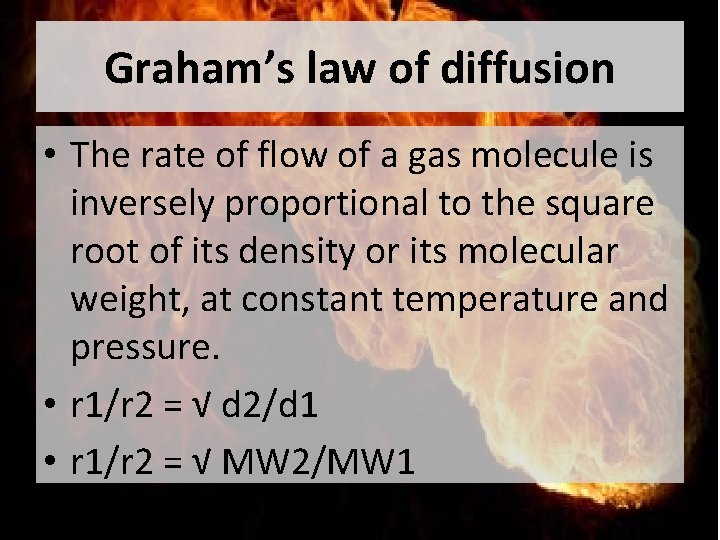Graham’s law of diffusion • The rate of flow of a gas molecule is inversely proportional to the square root of its density or its molecular weight, at constant temperature and pressure. • r 1/r 2 = √ d 2/d 1 • r 1/r 2 = √ MW 2/MW 1Example • How much faster does H 2 travel than O 2 molecule at the same temperature and pressure if molecular weights of H 2 and O 2 are 2 g/mole and 32 g/mole respectively? • H 2 molecules travel 4 times faster than O 2 molecules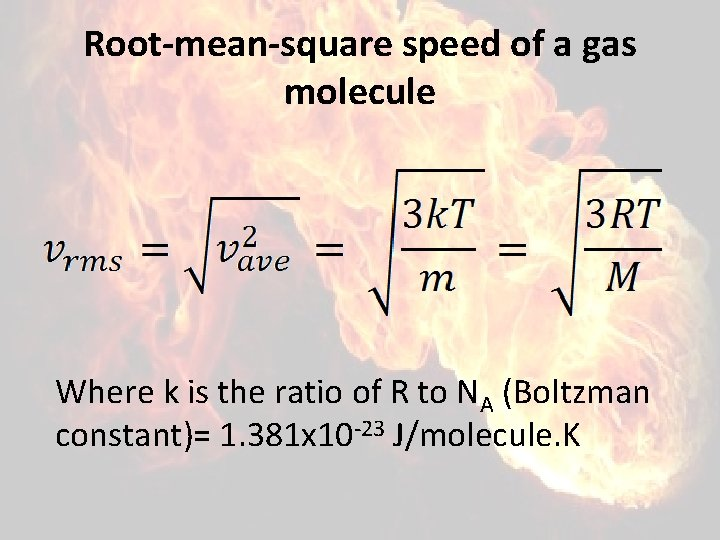Root-mean-square speed of a gas molecule Where k is the ratio of R to NA (Boltzman constant)= 1. 381 x 10 -23 J/molecule. K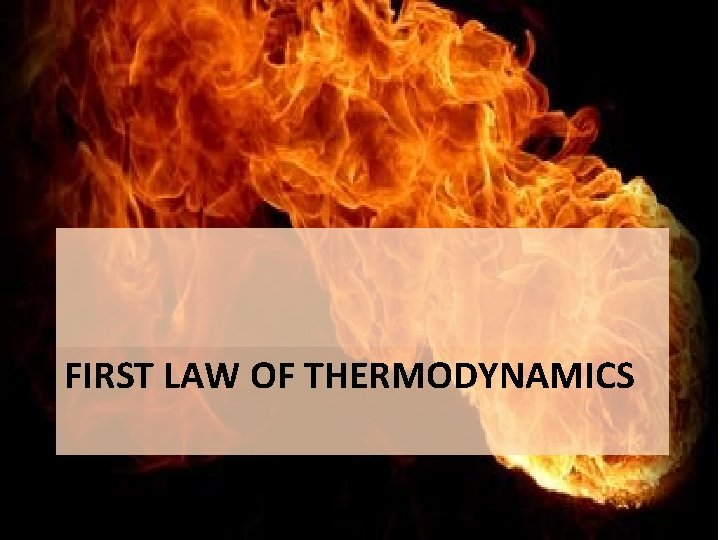FIRST LAW OF THERMODYNAMICSFirst Law of Thermodynamics Internal energy is the change in initial and final energies of the system Internal energy is the sum of heat exchange between the system and the surroundings and W done on or by the systemSign Convention • W done on the system: + • W done by the system: • Heat added to the system: + • Heat released by the system: -First Law of Thermodynamics Internal energy is the change in initial and final energies of the system Internal energy is the sum of heat exchange between the system and the surroundings and W done on or by the system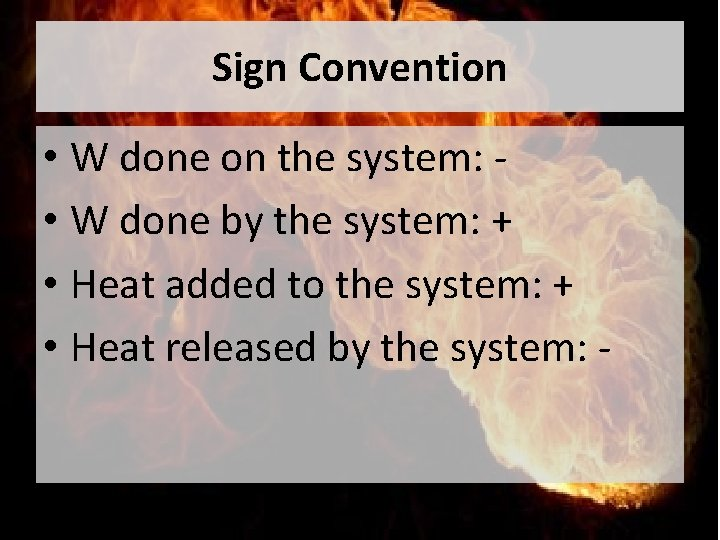Sign Convention • W done on the system: • W done by the system: + • Heat added to the system: + • Heat released by the system: -Heat engines, Internal-Combustion Engines, Refrigerators, Carnot Cycle, Entropy SECOND LAW OF THERMODYNAMICSSecond Law of Thermodynamics • It is a general principle which places constraints upon the direction of heat transfer and the attainable efficiencies of heat engines. • In so doing, it goes beyond the limitations imposed by the first law of thermodynamics.Second Law of Thermodynamics: heat engines • This is sometimes called the "first form" of the second law, and is referred to as the Kelvin. Planck statement of the second law. • It is impossible to extract an amount of heat QH from a hot reservoir and use it all to do work W. Some amount of heat QC must be exhausted to a cold reservoir.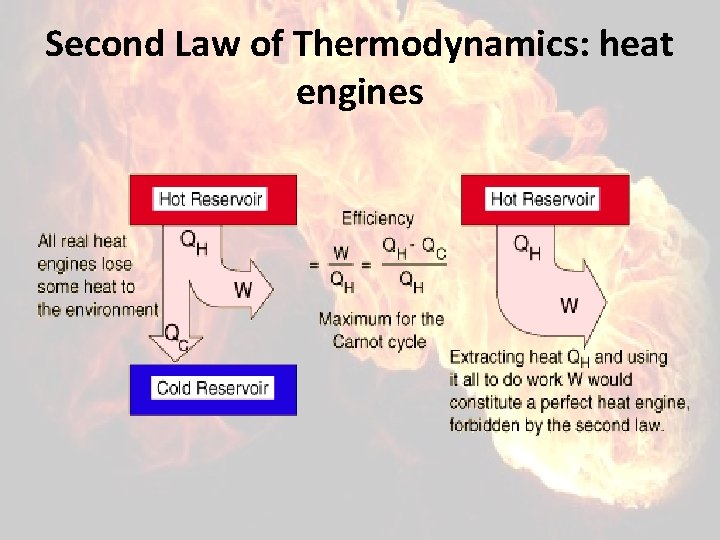Second Law of Thermodynamics: heat enginesSecond Law of Thermodynamics: heat engines • The most efficient heat engine cycle is the Carnot cycle, consisting of two isothermal processes and two adiabatic processes. • the Carnot efficiency: the processes involved in the heat engine cycle must be reversible and involve no change in entropy.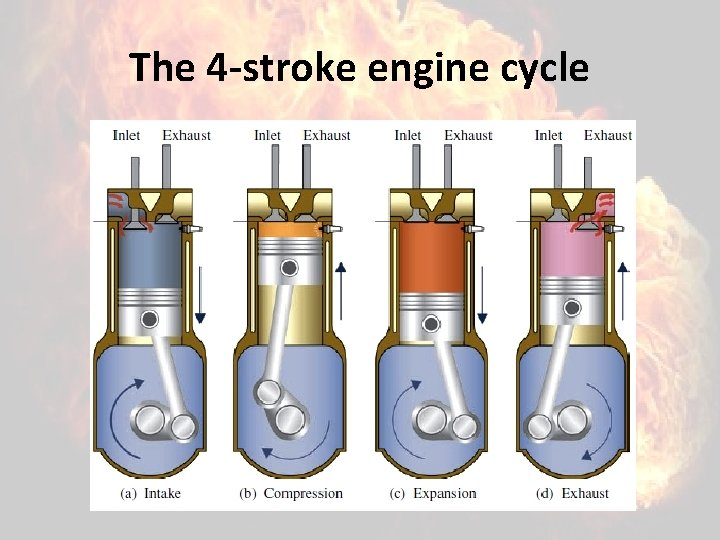The 4 -stroke engine cycleThe 4 -stroke engine cycle • Intake stroke: the inlet valve is open and a fresh fuel-air mixture is pulled into the cylinder by the downward motion of the piston.The 4 -stroke engine cycle • Compression stroke: the piston moves upward, compressing the mixture. The temperature and pressure increase. Prior to the piston reaching the top of its travel, the spark plug ignites the mixture and a flame begins to propagate across the combustion chamber.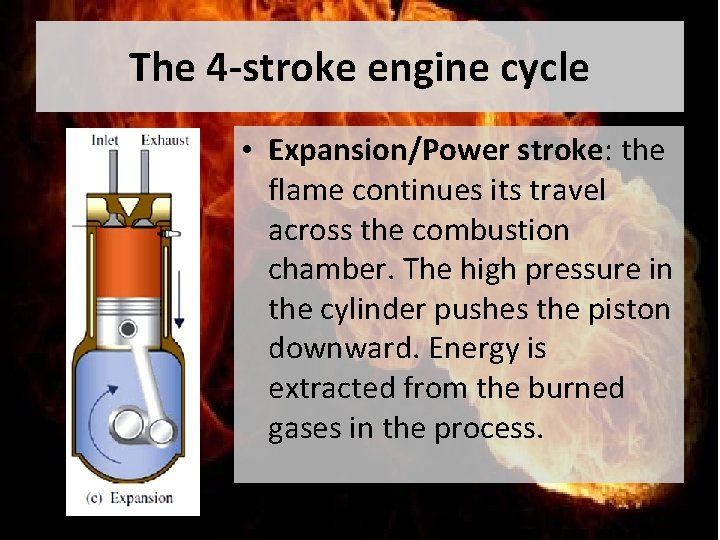The 4 -stroke engine cycle • Expansion/Power stroke: the flame continues its travel across the combustion chamber. The high pressure in the cylinder pushes the piston downward. Energy is extracted from the burned gases in the process.The 4 -stroke engine cycle • Exhaust stroke: the hot products flow rapidly out of the cylinder because of the relatively high pressure within the cylinder compared to that in the exhaust port.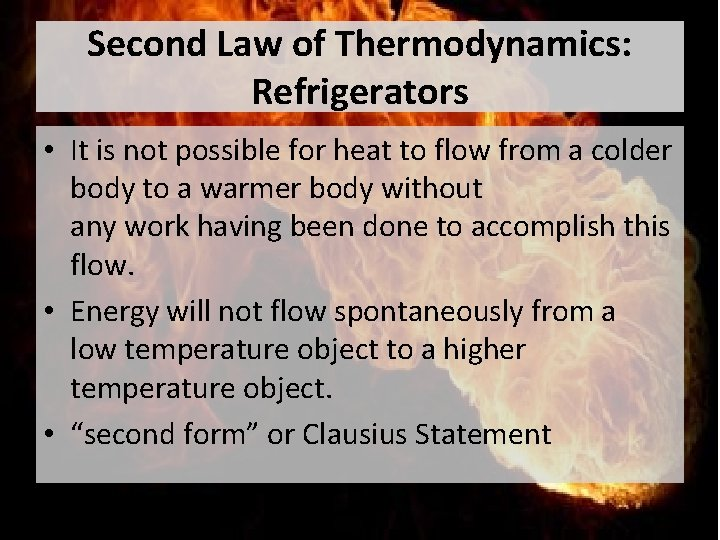Second Law of Thermodynamics: Refrigerators • It is not possible for heat to flow from a colder body to a warmer body without any work having been done to accomplish this flow. • Energy will not flow spontaneously from a low temperature object to a higher temperature object. • “second form” or Clausius Statement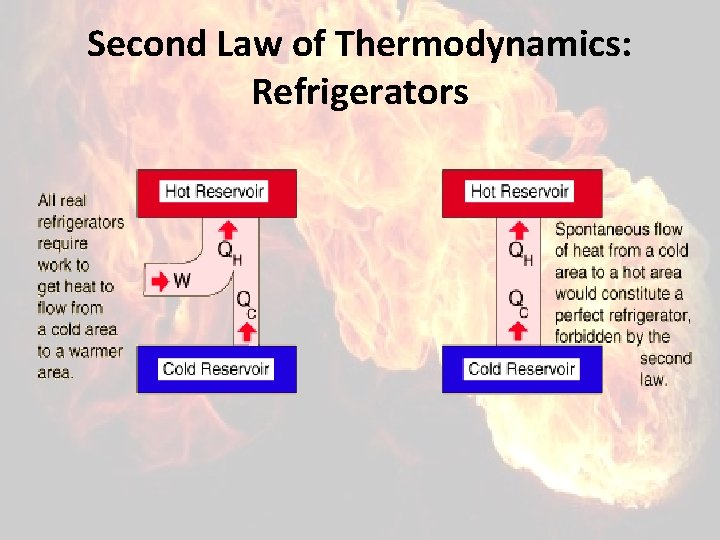Second Law of Thermodynamics: Refrigerators2 vs 7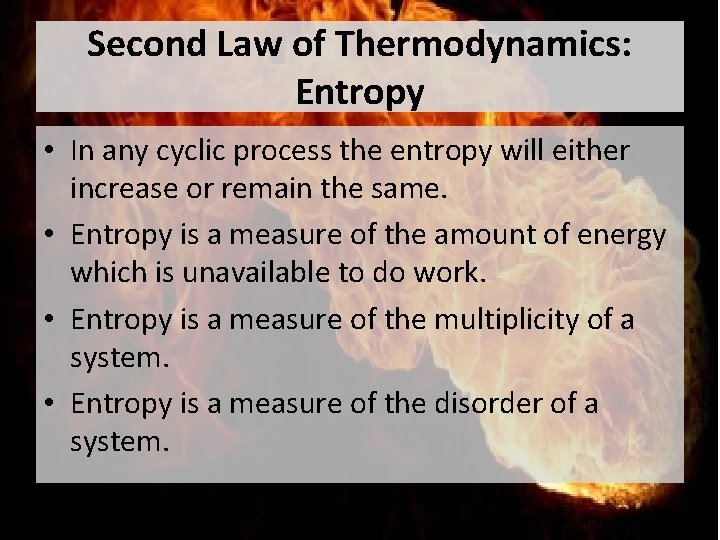Second Law of Thermodynamics: Entropy • In any cyclic process the entropy will either increase or remain the same. • Entropy is a measure of the amount of energy which is unavailable to do work. • Entropy is a measure of the multiplicity of a system. • Entropy is a measure of the disorder of a system. http: //hyperphysics. phy-astr. gsu. edu/hbase/thermo/seclaw. html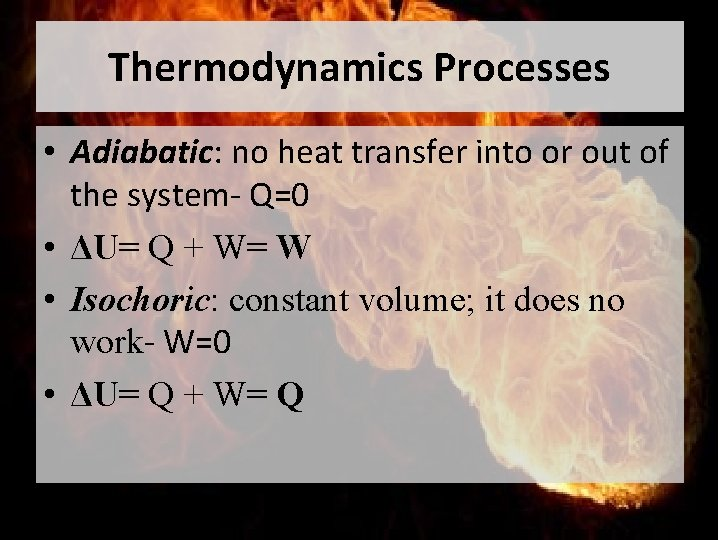Thermodynamics Processes • Adiabatic: no heat transfer into or out of the system- Q=0 • ΔU= Q + W= W • Isochoric: constant volume; it does no work- W=0 • ΔU= Q + W= QThermodynamics Processes • Isobaric: constant pressure • W= p(V 2 -V 1) • Isothermal: constant temperature- ΔU=0 • Q=W